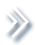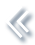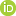####ArchivesReview Articles

## Rejoinder of “On studying extreme values and systematic risks with nonlinear time series models and tail dependence measures”

Zhengjun Zhangzjz@stat.wisc.edu

Pages 45-48 | Received 01 Jan. 2021, Accepted 01 Jan. 2021, Published online: 12 Jan. 2021,
• Abstract
• References
• Citations

## References

1. Brown, B. M., & Resnick, S. I. (1977). Extreme values of independent stochastic processes. Journal of Applied Probability14(4), 732–739. https://doi.org/10.2307/3213346 [Crossref][Web of Science ®], [Google Scholar]
2. Cao, W., & Zhang, Z. (2020). New extreme value theory for maxima of maxima. Statistical Theory and Related Fields. https://doi.org/10.1080/24754269.2020.1846115. [Taylor & Francis Online], [Google Scholar]
3. Davis, R. A., & Mikosch, T. (2009). The extremogram: A correlogram for extreme events. Bernoulli15(4), 977–1009. https://doi.org/10.3150/09-BEJ213 [Crossref][Web of Science ®], [Google Scholar]
4. Davis, R. A., & Resnick, S. I. (1989). Basic properties and prediction of max-ARMA processes. Advances in Applied Probability21, 781–803. https://doi.org/10.2307/1427767 [Crossref][Web of Science ®], [Google Scholar]
5. Davis, R. A., & Resnick, S. I. (1993). Prediction of stationary max-stable processes. The Annals of Applied Probability3(2), 497–525. https://doi.org/10.1214/aoap/1177005435 [Crossref], [Google Scholar]
6. Deng, L., Yu, M., & Zhang, Z. (2020). Statistical learning of the worst regional smog extremes with dynamic conditional modeling. Atmosphere11(665). https://doi.org/10.3390/atmos11060665 [Google Scholar]
7. Feng, W., Wang, Y., & Zhang, Z. (2018). Can cryptocurrencies be a safe haven: A tail risk perspective analysis. Applied Economics50(44), 4745–4762. https://doi.org/10.1080/00036846.2018.1466993 [Taylor & Francis Online][Web of Science ®], [Google Scholar]
8. Hall, P. (1982). On estimating the endpoint of a distribution. The Annals of Statistics10(2), 556–568. https://doi.org/10.1214/aos/1176345796 [Crossref][Web of Science ®], [Google Scholar]
9. Idowu, T., & Zhang, Z. (2017). An extended sparse max-linear moving model with application to high-frequency financial data. Statistical Theory and Related Fields1(1), 92–111. https://doi.org/10.1080/24754269.2017.1346852 [Taylor & Francis Online], [Google Scholar]
10. Ji, J., & Li, D. (2021). Application of autoregressive tail-index model to china's stock market. Statistical Theory and Related Fields. Accepted. [Taylor & Francis Online], [Google Scholar]
11. Joe, H. (2014). Dependence modeling with copulasChapman & Hall/CRC monographs on statistics & applied probability. Chapman & Hall/CRC. [Crossref], [Google Scholar]
12. Kabluchko, Z., Schlather, M., & de Haan, L. (2009). Stationary max-stable fields associated to negative definite functions. The Annals of Probability37(5), 2042–2065. https://doi.org/10.1214/09-AOP455 [Crossref][Web of Science ®], [Google Scholar]
13. Kunihama, T., Omori, Y., & Zhang, Z. (2012). Bayesian estimation and particle filter for max-stable processes. Journal of Time Series Analysis33(1), 61–80. https://doi.org/10.1111/jtsa.2011.33.issue-1 [Crossref][Web of Science ®], [Google Scholar]
14. Ledford, A. W., & Tawn, J. A. (1996). Statistics for near independence in multivariate extreme values. Biometrika83(1), 169–187. https://doi.org/10.1093/biomet/83.1.169 [Crossref][Web of Science ®], [Google Scholar]
15. Ledford, A. W., & Tawn, J. A. (1997). Modeling dependence within joint tail regions. Journal of the Royal Statistical Society: Series B (Statistical Methodology)59(2), 475–499. https://doi.org/10.1111/rssb.1997.59.issue-2 [Crossref], [Google Scholar]
16. Mao, G., & Zhang, Z. (2018). Stochastic tail index model for high frequency financial data with bayesian analysis. Journal of Econometrics205(2), 470–487. https://doi.org/10.1016/j.jeconom.2018.03.019 [Crossref][Web of Science ®], [Google Scholar]
17. McNeil, A. J., & Frey, R. (2000). Estimation of tail-related risk measures for heteroscedastic financial time series: An extreme value approach. Journal of Empirical Finance7(3–4), 271–300. https://doi.org/10.1016/S0927-5398(00)00012-8 [Crossref], [Google Scholar]
18. Naveau, P., Zhang, Z., & Zhu, B. (2011). An extension of max autoregressive models. Statistics and Its Interface4(2), 253–266. https://doi.org/10.4310/SII.2011.v4.n2.a19 [Crossref][Web of Science ®], [Google Scholar]
19. Oesting, M., Schlather, M., & Friederichs, P. (2017). Statistical post-processing of forecasts for extremes using bivariate Brown-Resnick processes with an application to wind gusts. Extremes20, 309–332. https://doi.org/10.1007/s10687-016-0277-x [Crossref][Web of Science ®], [Google Scholar]
20. Peng, L., & Qi, Y. (2009). Maximum likelihood estimation of extreme value index for irregular cases. Journal of Statistical Planning and Inference139(9), 3361–3376. https://doi.org/10.1016/j.jspi.2009.03.012 [Crossref][Web of Science ®], [Google Scholar]
21. Qi, Y. (2021). Discussion on paper “on studying extreme values and systematic risks with nonlinear time series models and tail dependence measures” by zhengjun zhang. Statistical Theory and Related Fields. Accepted. [Taylor & Francis Online], [Google Scholar]
22. Resnick, S. I. (2004). The extremal dependence measure and asymptotic independence. Stochastic Models20(2), 205–227. https://doi.org/10.1081/STM-120034129 [Taylor & Francis Online][Web of Science ®], [Google Scholar]
23. Schlather, M. (2002). Models for stationary max-stable random fields. Extremes5, 33–44. https://doi.org/10.1023/A:1020977924878 [Crossref], [Google Scholar]
24. Smith, R. L. (2021). Multivariate extremes and max-stable processes: Discussion of the paper by Zhengjun Zhang. Statistical Theory and Related Fields. Accepted. [Taylor & Francis Online], [Google Scholar]
25. Smith, R. L., & Weissman, I. (1996). Characterization and estimation of the multivariate extremal index (Technical report). University of North Carolina-Chapel Hill. [Google Scholar]
26. Tang, R., Shao, J., & Zhang, Z. (2013). Sparse moving maxima models for tail dependence in multivariate financial time series. Journal of Statistical Planning and Inference143(5), 882–895. https://doi.org/10.1016/j.jspi.2012.11.008 [Crossref][Web of Science ®], [Google Scholar]
27. Wang, F., Peng, L., Qi, Y., & Xu, M. (2019). Maximum penalized likelihood estimation for the endpoint and exponent of a distribution. Statistica Sinica29, 203–224. [Web of Science ®], [Google Scholar]
28. Wang, T., & Yan, J. (2021). Discussion of “on studying extreme values and systematic risks with nonlinear time series models and tail dependence measures”. Statistical Theory and Related Fields. Accepted. [Taylor & Francis Online], [Google Scholar]
29. Xu, W., & Wang, H. J. (2021). Discussion on “on studying extreme values and systematic risks with nonlinear time series models and tail dependence measures”. Statistical Theory and Related Fields. Accepted. [Taylor & Francis Online], [Google Scholar]
30. Yang, X., Frees, E. W., & Zhang, Z. (2011). A generalized beta copula with applications in modeling multivariate long-tailed data. Insurance: Mathematics and Economics49(2), 265–284. [Crossref][Web of Science ®], [Google Scholar]
31. Zhang, T. (2021a). Discussion of “on studying extreme values and systematic risks with nonlinear time series models and tail dependence measures”. Statistical Theory and Related Fields. Accepted. [Google Scholar]
32. Zhang, T. (2021b). High quantile regression for tail dependent time series. Biometrika. Forthcoming. [Crossref], [Google Scholar]
33. Zhang, Z. (2008). Quotient correlation: A sample based alternative to Pearson's correlation. The Annals of Statistics36(2), 1007–1030. https://doi.org/10.1214/009053607000000866 [Crossref][Web of Science ®], [Google Scholar]
34. Zhang, Z. (2020). On studying extreme values and systematic risks with nonlinear time series models and tail dependence measures (with discussions). Statistical Theory and Related Fields. https://doi.org/10.1080/24754269.2020.1856590 [Taylor & Francis Online], [Google Scholar]
35. Zhang, Z., Qi, Y., & Ma, X. (2011). Asymptotic independence of correlation coefficients with application to testing hypothesis of independence. Electronic Journal of Statistics5, 342–372. https://doi.org/10.1214/11-EJS610 [Crossref][Web of Science ®], [Google Scholar]
36. Zhang, Z., & Smith, R. L. (2004). The behavior of multivariate maxima of moving maxima processes. Journal of Applied Probability41(4), 1113–1123. https://doi.org/10.1239/jap/1101840556 [Crossref][Web of Science ®], [Google Scholar]
37. Zhang, Z., & Smith, R. L. (2010). On the estimation and application of max-stable processes. Journal of Statistical Planning and Inference140(5), 1135–1153. https://doi.org/10.1016/j.jspi.2009.10.014 [Crossref][Web of Science ®], [Google Scholar]
38. Zhang, Z., & Zhu, B. (2016). Copula structured m4 processes with application to high-frequency financial data. Journal of Econometrics194(2), 231–241. https://doi.org/10.1016/j.jeconom.2016.05.004 [Crossref][Web of Science ®], [Google Scholar]
39. Zhao, Z., & Zhang, Z. (2018). Semi-parametric dynamic max-copula model for multivariate time series. Journal of the Royal Statistical Society, Series B80, 409–432. https://doi.org/10.1111/rssb.12256 [Crossref], [Google Scholar]
40. Zhao, Z., Zhang, Z., & Chen, R. (2018). Modeling maxima with autoregressive conditional Fréchet model. Journal of Econometrics207, 325–351. https://doi.org/10.1016/j.jeconom.2018.07.004 [Crossref][Web of Science ®], [Google Scholar]